/

### Blending Independent Components and Principal Components Analysis 4.2 Different blended importance criteria

Next page

4.2          Different blended importance criteria

One possible approach would be to use an importance criterion involving a function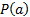that includes both variance and factors like kurtosis that characterise the extent to which the data seems to be coming from a non-normal distribution. For example, we might use the following: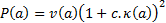(N.B. We could also use any function that varied monotonically in line with this function, e.g. its square root , ifis positive, and we would derive exactly the same input signals)

Here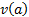is the variance of the time series corresponding to the mixture of output signals characterised by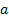,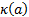is its kurtosis, andis a constant that indicates the extent to which we want to focus on kurtosis rather than variance in the derivation of which signals might be ‘important’. Again we would constrainto be of ‘unit length’, i.e. to have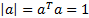.

The larger (i.e. more positive)is, the more we might expect such an approach to tend to highlight signals that exhibit positive kurtosis. Thus the closer the computed unmixed input signals should be to those that would be derived by applying ICA to the mixed signals (if the ICA was formulated using model pdfs with high kurtosis). We here need to assume thatdoes not vary ‘too much’ with respect to, so that in the limit as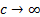any signal exhibiting suitably positive kurtosis will be selected at some stage in the iterative process, although we might expect variation into ‘blur’ together some signals that ICA might otherwise distinguish. The smaller (i.e. closer to zero)is, the closer the result should be to a PCA analysis.

However, there are several possible weaknesses with such an approach:

(a)    There is no immediately obvious reason to choose any particular value of. This is because we have not introduced into the problem specification any particular relative importance to ascribe to variance versus kurtosis. One possible solution to this problem is to focus application of such a methodology onto a problem that does potentially provide some guidance in this area. The most obvious such application would be portfolio risk measurement in a situation where we wanted to measure risk not by reference to variance of relative return (or a monotonically equivalent measure such as standard deviation) but by reference to some other metric such as Value-at-Risk or Expected Shortfall that places greater weight on tail behaviour. We could for example ‘extrapolate’ into the tail based on observed variance and kurtosis (and also skew) using the 4th order Cornish Fisher asymptotic expansion. According to this expansion, we can estimate the quantile of a distribution relative to that which would apply were the distribution to have no skew or variance using the following formula, see e.g. Kemp (2009):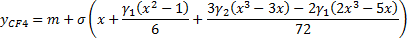Here,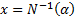,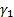is the skew of the distribution and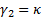is the kurtosis of the distribution, where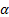is the probability to which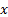applies and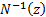is the inverse normal distribution function.

For example, we might adopt a 1 in 200 quantile cut-off, in which case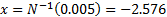. For a distribution with zero skew, we might thus apply an importance criterion that sought to maximise: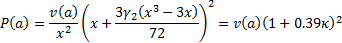The physical interpretation of this is that, if these assumptions apply, then the 1 in 200 quantile is a factor of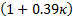further into the tail than we might otherwise expect purely from the standard deviation of the distribution.

(b)   Unfortunately, the 4th order Cornish-Fisher expansion is not in general very good at estimating the shape of the distributional form in regions in which we might be most interested, see e.g. Kemp (2009). In effect, the computation of skew and kurtosis gives ‘too much’ weight to the extent of non-normality in the centre of the distributional form whereas typically for risk management purposes we are most interested in the extent of non-normality in the tail of the distribution. He proposes an alternative approach, more directly akin to fitting a curve through the observed (ordered) distributional form, to ‘extrapolate into the tail’.

Such an approach is more computationally intensive than the Cornish-Fisher approach, particularly if the data series in question involve a large number of terms. The approach requires the return series to be sorted, in order to work out which observations to give most weight to in the curve fitting algorithm. Sorting large data sets is intrinsically much slower than merely calculating their moments since it typically involves a number of computations that scales in line with approximately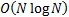rather than merely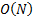. It may be that such a refinement would not in practice lead to a much enhanced risk model, as non-zero kurtosis is still typically a good indicator of the presence of fat-tailed behaviour, even if it is not a particularly good indicator of exactly how fat-tailed it is in the particular part of the distributional form in which we might be most interested.

(c)    More problematic, perhaps, is another topic that Kemp explores in Kemp (2009) and Kemp (2010). He notes, as implicitly have earlier authors, that much of the fat-tailed behaviour observed in practice in return series (both when viewed singly and when viewed jointly) seems to derive from time-varying volatility, see Section 4.3.